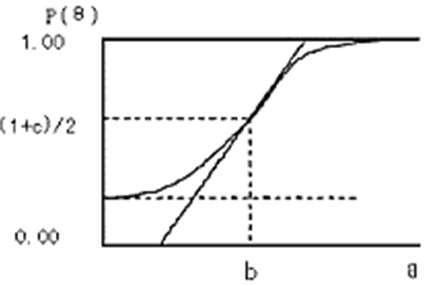# IRT模型学习小结

## 关于IRT模型

1. 能力与观测分数之间的线性关系假设不合理。
在经典测量理论中认为被试的能力与测试得到的分数是线性关系的，但是在实际情况中往往不程线性关系。比如我们在进行考试时，想要从50分考到60分很容易，但是想要从90分考到100分却很难。
2. 被试的测验结果依赖于所施测项目的难度。
被试由于做难易不同的题目而得到不同的分数，在经典测量理论中不同的分数表示不同的能力，这与常理不符。被试的能力不应由于所测题目的难度不同而不同，即，被试的能力应于所测题目的难度无关。
3. 无法提供能力不同的被试如何对项目做出反应。
对于已知能力的被试以及已知相关参数的题目，经典测量理论无法给出被试做该道题目的正确或错误概率

1. 参数具有不变性。
在项目反应理论下，项目的难度参数、区分度参数及被试的能力参数具有不变性。
2. 被试的能力水平与项目参数之间关联化、模型化。
在 IRT 中引入了项目特征曲线，这将项目难度、项目区分度以及被试的能力进行了有机的统一。
3. IRT 定义了信息函数。
IRT 中的信息函数反映了在不同的能力水平处，每个项目所提供的信息量的大小，信息量最大处的能力水平估计误差最小。
4. 被试的能力参数与项目的难度参数具有配套性。
被试的能力参数与项目的难度参数是定义在同一个量表上的，当一个被试的能力参数已知时，配一个项目参数已知的测验，即可预测被试的正确反应概率。

# IRT模型原理

## 模型介绍

IRT模型是用于评估被试对某一项目或某一类项目的潜在特质。
IRT模型是建立在一定的假设之下：

• 单维性假设，即假设某个测验只测量被试的某一种能力。
• 立性假设，即假设被试在每一个项目上的作答反应是相互独立，互不影响的，作答反应只与被试自身的能力水平有关，与其他元素无关。
• 模型假设，即被试在项目上的正确反应概率与被试的能力水平有一定的函数关系。

IRT三参数logistic模型为：

p

i

(

θ

)

=

c

i

+

1

c

i

1

+

e

x

p

(

d

a

i

(

θ

b

i

)

)

p_i(\theta)=c_i+\frac{1-c_i}{1+exp(-da_i(\theta-b_i))}

pi(θ)=ci+1+exp(dai(θbi))1cid 常数 1.702
a_i 项目的区分度系数 表示项目或题目的区分度，在曲线中影响曲线中部的斜率，当斜率越小，那么就很难将被试的测试分数结果区分开
b_i 项目的难度系数 表示项目或题目的难度，在曲线中代表曲线横轴方向的位移，难度系数越大，则被试想要获得比较高的分数就需要比较高的能力
c_i 项目的猜测系数 表示即使被试对测试的项目一点先验知识都没有，靠蒙也能蒙对的概率，比如选择题有0.25的概率才对

θ

\theta

p

i

(

θ

)

p_i(\theta)

a

i

a_i

ai

b

i

b_i

bi

c

i

c_i

ci，以及被试能力参数

θ

\theta

θ的估计。

## 参数估计

IRT模型的参数估计方法有很多，包括极大似然估计，EM算法，贝叶斯算法等，本文将简单介绍使用极大似然估计方法进行参数估计过程。

• 获取得分矩阵

User

i

t

e

m

1

item_1

i

t

e

m

2

item_2

i

t

e

m

3

item_3

i

t

e

m

M

item_M

u

1

u_1

1 0 1 1

u

2

u_2

0 0 1 0

u

3

u_3

1 1 1 0

u

N

u_N

0 1 1 1

u

n

u_n

un表示第n个被试，

i

t

e

m

m

item_m

itemm表示第m个项目，表格中的0表示被试n做错了项目m，1表示被试n做对了项目m。

• 参数估计
假设当项目的区分度系数，难度系数，猜测系数均已知时，将上面得分矩阵中的做题结果数据和已知的区分度系数，难度系数，猜测系数代入IRT模型中，建立能力参数的极大似然函数

L

=

i

=

1

m

p

i

y

i

(

1

p

i

)

1

y

i

L=\prod_{i=1}^{m}p_{i}^{y_i}(1-p_i)^{1-y_i}

式中，

p

i

p_i

即为IRT模型函数得到的答对概率，

y

i

y_i

即为上述得分矩阵中被试真实是否答对的标签。将该函数取对数，得到

l

n

(

L

)

=

i

=

1

m

y

i

l

n

(

p

i

)

+

(

1

y

i

)

l

n

(

1

p

i

)

ln(L)=\sum_{i=1}^{m}y_iln(p_{i})+(1-y_i)ln(1-p_i)

对上述对数极大似然函数进行求导，便可计算得到能力参数

θ

\theta

如果区分度系数，难度系数，猜测系数和能力系数均未知，则可为其中一方设置初始值，然后不断迭代计算出各个参数，或者建立联合极大似然函数进行估计。

# 应用场景

• 学习效果评估
在教育机构中，可以根据学生以往的做题情况，比如学生对某一知识点的相关题目的做题正确和错误情况数据，用IRT模型来估计出学生在该知识点上的能力值

θ

\theta

，从而了解学生的学习效果。

• 试卷的设计与修改
项目信息函数能够描述给处于某一能力值

θ

\theta

的被试提供一个测试或者一个项目的测量有效性。
项目信息函数

I

(

θ

,

μ

i

)

=

p

i

(

θ

)

2

p

i

(

θ

)

(

1

p

i

(

θ

)

)

I(\theta,\mu_i)=\frac{p_{i}'(\theta)^2}{p_i(\theta)(1-p_i(\theta))}

测试信息函数

I

(

θ

)

=

i

1

n

I

(

θ

,

μ

i

)

I(\theta)=\sum_{i-1}^{n}I(\theta, \mu_i)

测试信息函数是项目信息函数的累加。项目信息函数或测试信息函数值越大，表示该项目或测试对与该能力的学生能够反应越多的信息。据此来设计和修改得到更加有效的试卷或选择试题。

• 计算机自适应测试
在学生进行在线测试时，收集用户在线测试的实时信息来更新学生的能力

θ

\theta

，然后使用项目信息函数来选择更加有效的题目来提供给学生，从而达到更好的测试和学习效果

THE END